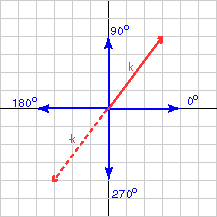```arc tan( y/x ) = arc tan( 4/3 ) = arc tan( 1.333333333333 ) =  53.13°
```

# Watch those "-" SignsThe diagram shows the vector represented by k = (3, 4)T. Its orientation was calculated to be 53.13° to the positive x axis. That looks about right. The formula worked!

Now calculate the orientation of     -k = (-3, -4)T.

Plugging into the formula:

```arc tan( y/x ) = arc tan( -4/-3 ) = arc tan( 4/3 )
= arc tan( 1.333333333333 )
=  53.13°
```

Hmm... something is wrong. The formula gave us the same angle for a vector pointing in the opposite direction to the first. The problem is that information is lost when -4 is divided by -3. We can't tell the result from +4 divided by +3. The formula is not enough to give you the answer; you should sketch the vector and adjust the answer.

Look at the picture to see that the orientation of -k (expressed in degrees 0..360 counter clockwise from the x axis) is (180° + 53.13°) = 233.13°.

### QUESTION 4:

What is the orientation of the vector represented by: p = (3,-4)T ?

Use the calculator application on your computer.